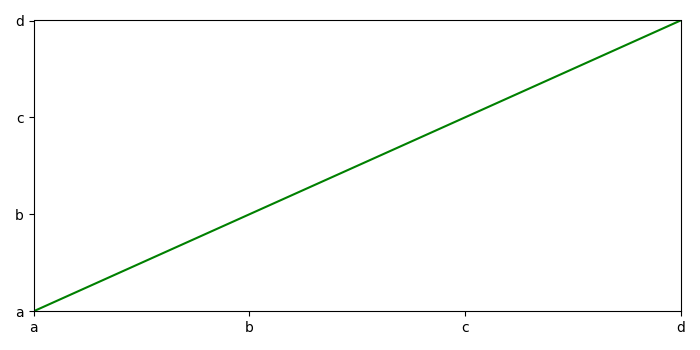# How to specify values on Y-axis in Python Matplotlib?

To specify values on Y-axis in Python, we can take the following steps−

• Create x and y data points using numpy.
• To specify the value of axes, create a list of characters.
• Use xticks and yticks method to specify the ticks on the axes with x and y ticks data points respectively.
• Plot the line using x and y, color=red, using plot() method.
• Make x and y margin 0.
• To display the figure, use show() method.

## Example

import numpy as np
from matplotlib import pyplot as plt
plt.rcParams["figure.figsize"] = [7.00, 3.50]
plt.rcParams["figure.autolayout"] = True
x = np.array([0, 2, 4, 6])
y = np.array([1, 3, 5, 7])
ticks = ['a', 'b', 'c', 'd']
plt.xticks(x, ticks)
plt.yticks(y, ticks)
plt.plot(x, y, c='green')
plt.margins(x=0, y=0)
plt.show()

## Output